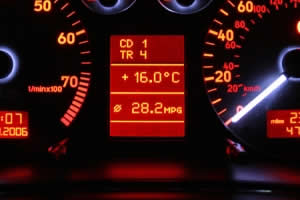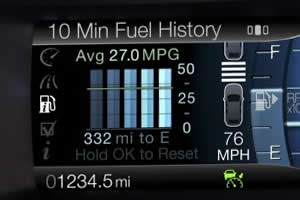# Mpg to kpl conversion formulae

## Miles per UK gallon to kilometres per litre conversion formula

To convert miles per UK gallon to kilometres per litre is fairly simple and can be done with a simple conversion factor, however it is easier to explain it as a two step process.

Step 1: Convert miles per UK gallon to kilometres per UK gallon by multiplying by 1.609, the conversion factor for miles to kilometres.

Step 2: Convert kilometres per UK gallon to kilometres per litre by dividing by 4.546 the conversion factor for litres to UK gallons.

ThUS to get kilometres per litre from miles per UK gallon the formula is:

kpl = (1.609 * mpg)/4.546

This can be simply expressed as kpl = 0.354 * mpg## Miles per US gallon to kilometres per litre conversion formula

To convert miles per US gallon to kilometres per litre follow the same steps as above but replace the 4.546 by 3.785 the conversion factor for litres to US gallons.

ThUS to get kilometres per litre from miles per US gallon the formula is:

kpl = (1.609 * mpg)/3.785

This can be simply expressed as kpl = 0.425 * mpg## Kilometres per litre to miles per UK gallon conversion formula

To convert kilometres per liter to miles per UK gallon is a simple as the previoUS conversions and again is easier to explain as a two step process.

First convert kilometres per litre to miles per litre by dividing by 1.609 (the conversion factor for miles to kilometres, as we are converting from kilometres to miles we need to divide by the conversion factor).

Then convert from miles per litre to miles per UK gallon by multiplying by 4.546, the conversion factor for litres to UK gallons.

ThUS to get miles per UK gallon from kilometres per litre the formula is:

mpg = (kpl/1.609) * 4.546

This can be simply expressed as mpg = 2.825 * kpl

## Kilometres per litre to miles per US gallon conversion formula

As with the miles per US gallon to kilometres per litre conversion converting from kilometres per litre to miles per US gallon can be done as per the above kilometres per litre to miles per UK gallon conversion, just change the 4.546 to 3.785, the conversion factor for litres to litres to US gallons.

ThUS to get miles per US gallon from kilometres per litre the formula is:

mpg = (kpl/1.609) * 3.785

This can be simply expressed as mpg = 2.352 * kpl